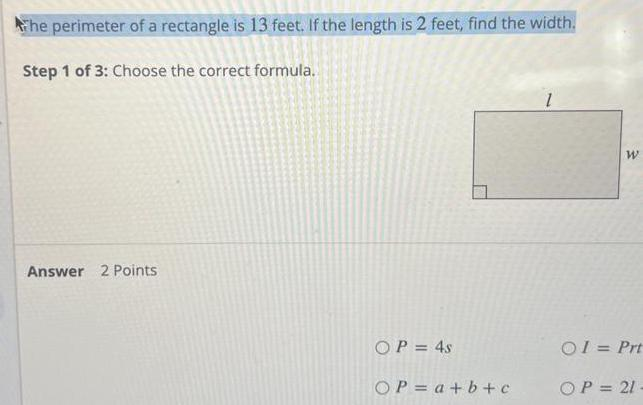Question:

# The perimeter of a rectangle is 13 feet If the length is 2

Last updated: 9/15/2023The perimeter of a rectangle is 13 feet If the length is 2 feet find the width Step 1 of 3 Choose the correct formula Answer 2 Points OP 4s OP a b c W 01 Prt OP 21